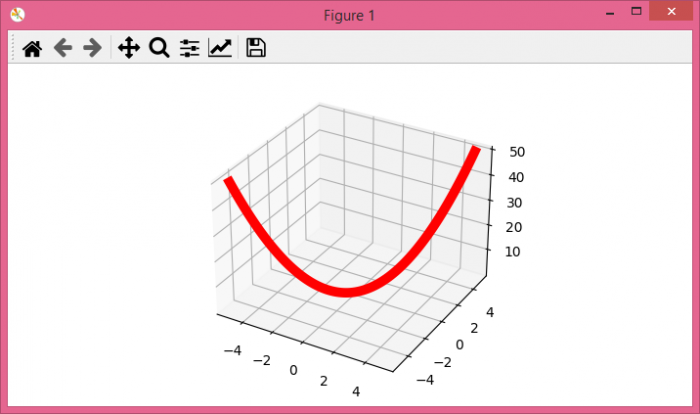# How to add a 3d subplot to a matplotlib figure?n

To add a 3D subplot to a matplotlib figure, we can take the following steps −

• Set the figure size and adjust the padding between and around the subplots.
• Create x, y and z data points using numpy.
• Create a new figure or activate an existing figure.
• Add an 'ax' to the figure as part of a subplot arrangement with projection='3d'.
• Plot x, y and z data points using plot() method.
• To display the figure, use .show() method.

## Example

from matplotlib import pyplot as plt
import numpy as np

# Set the figure size
plt.rcParams["figure.figsize"] = [7.00, 3.50]
plt.rcParams["figure.autolayout"] = True

# Create x, y and z data points using numpy
x = np.linspace(-5, 5, 100)
y = np.linspace(-5, 5, 100)
z = x ** 2 + y ** 2

fig = plt.figure()

plt.show()